# tensorflow自学之前的bigpicture

1、 tensorflow是什么？

tensorflow 是 google 开源的机器学习工具，在2015年11月其实现正式开源，开源协议Apache 2.0。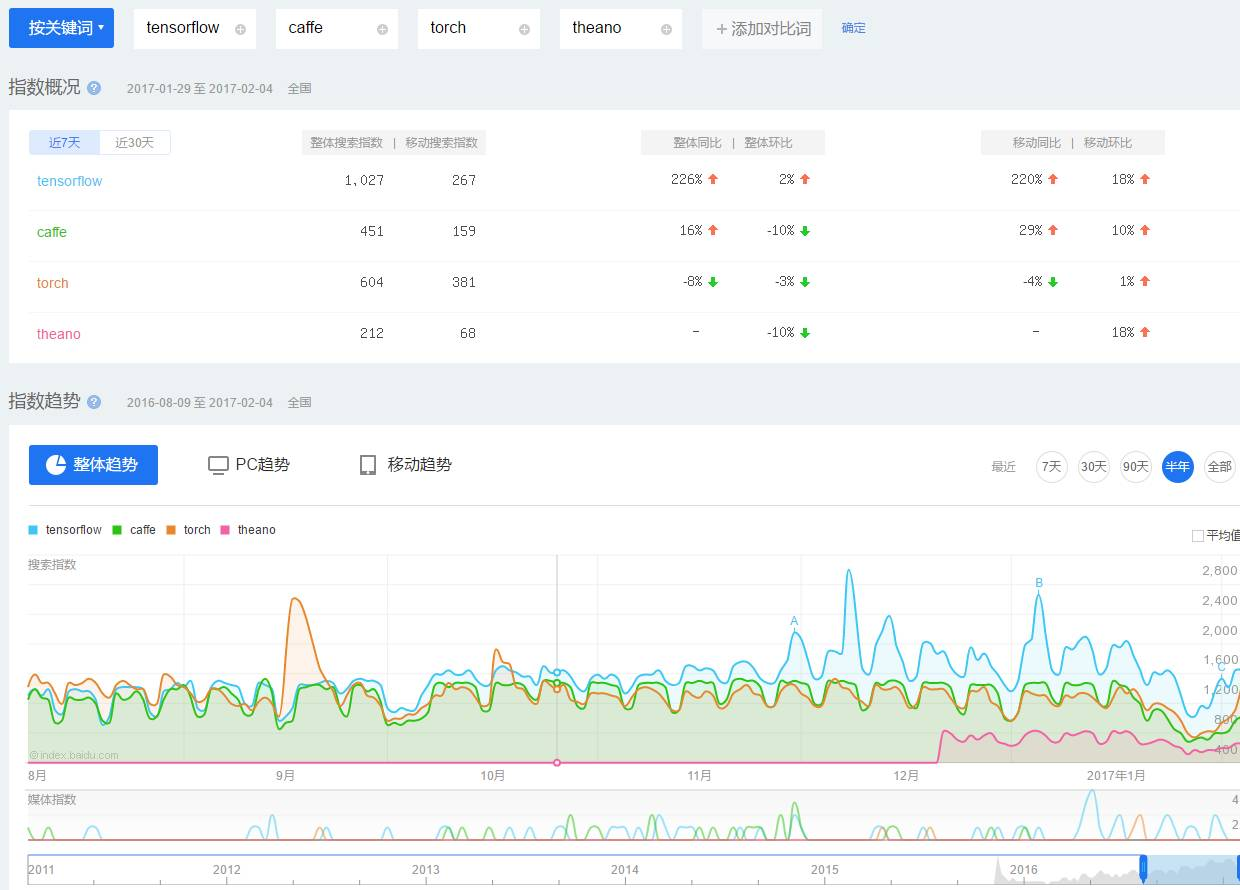2、 why tensorflow?

Tensorflow 拥有易用的 python 接口，而且可以部署在一台或多台 cpu , gpu 上，兼容多个平台，包括但不限于 安卓/windows/linux 等等平台上，而且拥有 tensorboard这种可视化工具，可以使用 checkpoint 进行实验管理，得益于图计算，它可以进行自动微分计算，拥有庞大的社区，而且很多优秀的项目已经使用 tensorflow 进行开发了。

3、 易用的tensorflow工具

4、 tensorflow安装

实际上编写tensorflow可以总结为两步.

（1）组装一个graph;

（2）使用session去执行graph中的operation。

因此我们从 graph 与 session 说起。

1、 graph与session

（1）计算图

Tensorflow 是基于计算图的框架，因此理解 graph 与 session 显得尤为重要。不过在讲解 graph 与 session 之前首先介绍下什么是计算图。假设我们有这样一个需要计算的表达式。该表达式包括了两个加法与一个乘法，为了更好讲述引入中间变量c与d。由此该表达式可以表示为：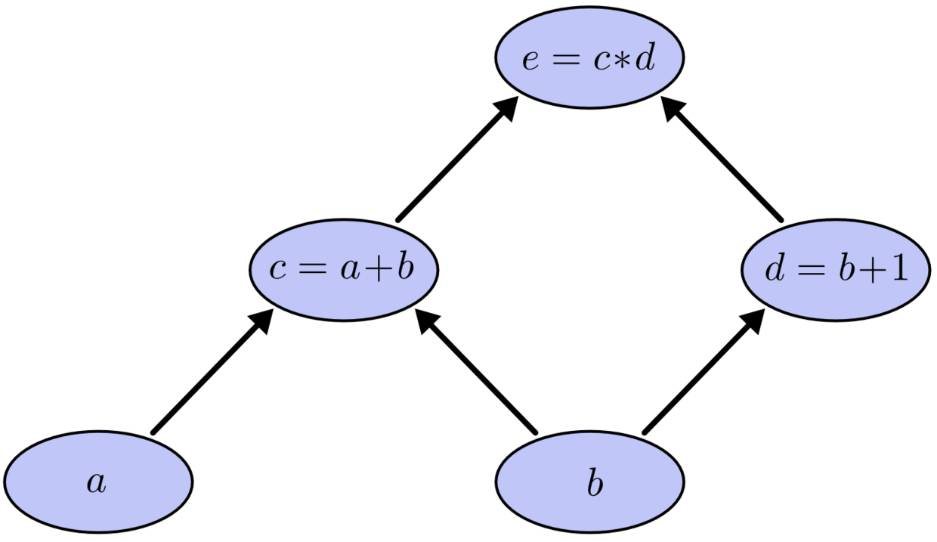（2）概念说明

Tensor：类型化的多维数组，图的边；

Operation:执行计算的单元，图的节点；

Graph：一张有边与点的图，其表示了需要进行计算的任务；

Session:称之为会话的上下文，用于执行图。

Graph仅仅定义了所有 operation 与 tensor 流向，没有进行任何计算。而session根据 graph 的定义分配资源，计算 operation，得出结果。既然是图就会有点与边，在图计算中 operation 就是点而 tensor 就是边。Operation 可以是加减乘除等数学运算，也可以是各种各样的优化算法。每个 operation 都会有零个或多个输入，零个或多个输出。 tensor 就是其输入与输出，其可以表示一维二维多维向量或者常量。而且除了Variables指向的 tensor 外所有的 tensor 在流入下一个节点后都不再保存。

（3）举例

import tensorflow as tf
graph = tf.Graph()
with graph.as_default():
foo = tf.Variable(3,name='foo')
bar = tf.Variable(2,name='bar')
result = foo + bar
initialize = tf.global_variables_initializer()

print(result)  #Tensor("add:0", shape=(), dtype=int32)

print(result)  #Tensor("add:0", shape=(), dtype=int32)

with tf.Session(graph=graph) as sess:
sess.run(initialize)
res = sess.run(result)
print(res)  # 5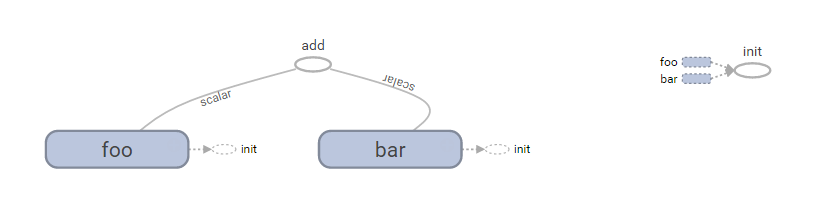2、数据结构

Tensorflow的数据结构有着rank,shape,data types的概念，下面来分别讲解。

（1）rank

Rank一般是指数据的维度，其与线性代数中的rank不是一个概念。其常用rank举例如下。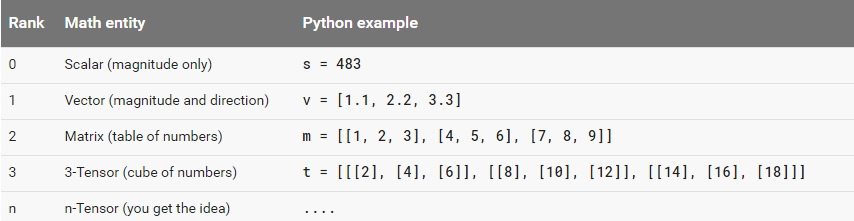（2）shape

Shape指tensor每个维度数据的个数，可以用python的list/tuple表示。下图表示了rank,shape的关系。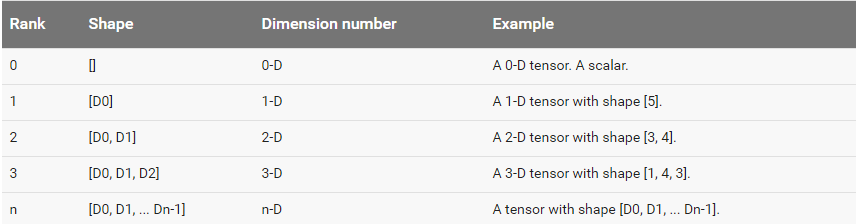（3）data type

Data type，是指单个数据的类型。常用DT_FLOAT，也就是32位的浮点数。下图表示了所有的types。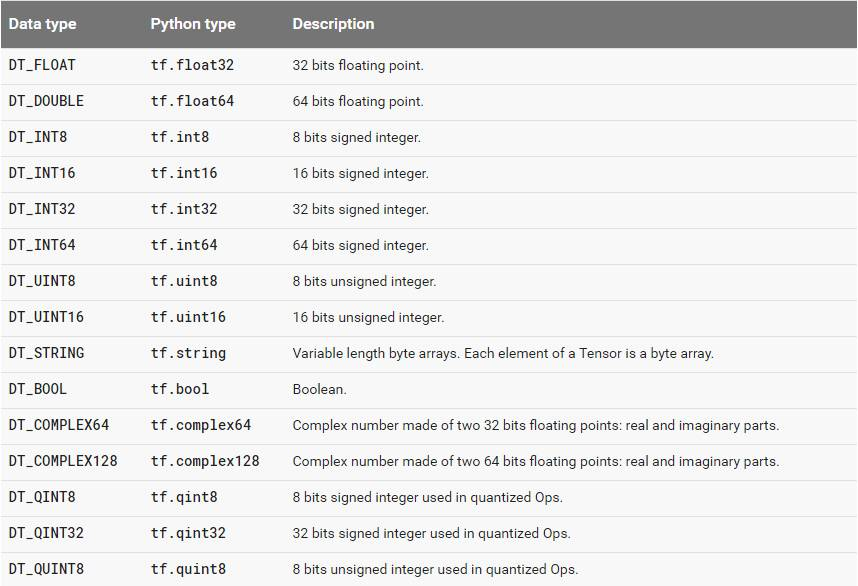3、 Variables

（1）介绍

weights = tf.Variable(tf.random_normal([784, 200], stddev=0.35),name="weights")
biases = tf.Variable(tf.zeros(), name="biases")

（2）初始化

Init_ab = tf.variables_initializer([a,b],name=”init_ab”)
Variables可以通过eval显示其值，也可以通过assign进行赋值。Variables支持很多数学运算，具体可以参照官方文档

（3）Variables与constant的区别

const = tf.constant(1.0,name="constant")
print(tf.get_default_graph().as_graph_def())

node {
name: "constant"
op: "Const"
attr {
key: "dtype"
value {
type: DT_FLOAT
}
}
attr {
key: "value"
value {
tensor {
dtype: DT_FLOAT
tensor_shape {
}
float_val: 1.0
}
}
}
}
versions {
producer: 17
}

（4）命名

4、 placeholders与feed_dict

foo = tf.placeholder(tf.int32,shape=,name='foo')
bar = tf.constant(2,name='bar')
result = foo + bar
with tf.Session() as sess:
print(sess.run(result)) 

print(sess.run(result,{foo:}))

from tensorflow.examples.tutorials.mnist import input_data
mnist = input_data.read_data_sets("MNIST_data/", one_hot=True)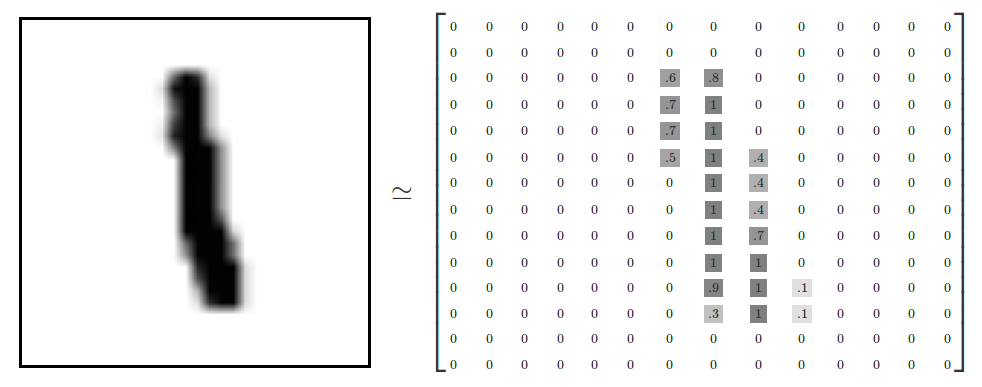y为X真实的类别，其数据可以看做如下图的形式。因此，问题可以看成一个10分类的问题：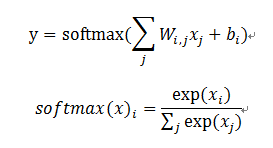1、为输入X输出y定义placeholder；

2、定义权重W；

3、定义模型结构；

4、定义损失函数；

5、定义优化算法。

import tensorflow as tf
x = tf.placeholder(tf.float32, [None, 784])
y_ = tf.placeholder(tf.float32, [None, 10])
W = tf.Variable(tf.zeros([784, 10]))
b = tf.Variable(tf.zeros())


y = tf.nn.softmax(tf.matmul(x, W) + b)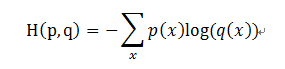cross_entropy = tf.reduce_mean(-tf.reduce_sum(y_*tf.log(y),reduction_indices=))
train_step = tf.train.GradientDescentOptimizer(0.5).minimize(cross_entropy)
init = tf.global_variables_initializer()

with tf.Session() as sess:
sess.run(init)
for i in range(1000):
batch_xs, batch_ys = mnist.train.next_batch(100)
sess.run(train_step, feed_dict={x:  batch_xs, y_: batch_ys})

correct_prediction = tf.equal(tf.argmax(y,1), tf.argmax(y_,1))
accuracy = tf.reduce_mean(tf.cast(correct_prediction, tf.float32))
print(sess.run(accuracy, feed_dict={x: mnist.test.images, y_: mnist.test.labels}))